• import open3d as o3d print("绘制立方体") mesh_box = o3d.geometry.TriangleMesh.create_box(width=1.0python
• python表白玫瑰花绘制——情人节...python表白玫瑰花绘制——情人节表白python表白玫瑰花绘制——情人节表白一、玫瑰花绘制一二、玫瑰花绘制二三、玫瑰花绘制三四、桃花绘制 一、玫瑰花绘制一 fig = plt.figure() a
python表白玫瑰花绘制——情人节表白
搬运不易，路过的各位大佬请点个赞

python表白玫瑰花绘制——情人节表白
python表白玫瑰花绘制——情人节表白一、玫瑰花绘制—深红色二、玫瑰花绘制—五颜六色三、玫瑰花绘制—粉红色四、玫瑰花绘制—红色五、桃花绘制

一、玫瑰花绘制—深红色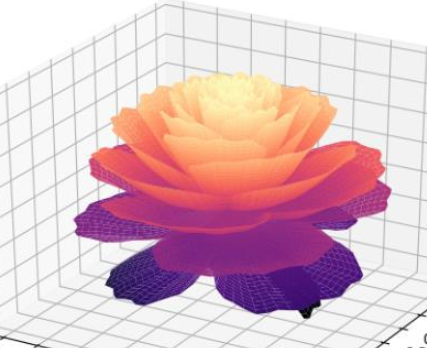import numpy as np
import matplotlib.pyplot as plt
from mpl_toolkits.mplot3d import Axes3D
fig = plt.figure()
ax = fig.gca(projection='3d')
[x, t] = np.meshgrid(np.array(range(25)) / 24.0, np.arange(0, 575.5, 0.5) / 575 * 30 * np.pi - 4*np.pi)
p = (np.pi / 2) * np.exp(-t / (8 * np.pi))
change = np.sin(20*t)/50
u = 1 - (1 - np.mod(3.3 * t, 2 * np.pi) / np.pi) ** 4 / 2 + change
y = 2 * (x ** 2 - x) ** 2 * np.sin(p)
r = u * (x * np.sin(p) + y * np.cos(p)) * 1.5
h = u * (x * np.cos(p) - y * np.sin(p))
c= plt.get_cmap('magma')
surf = ax.plot_surface(r * np.cos(t), r * np.sin(t), h, rstride=1, cstride=1,
cmap= c, linewidth=0, antialiased=True)
plt.show()


二、玫瑰花绘制—五颜六色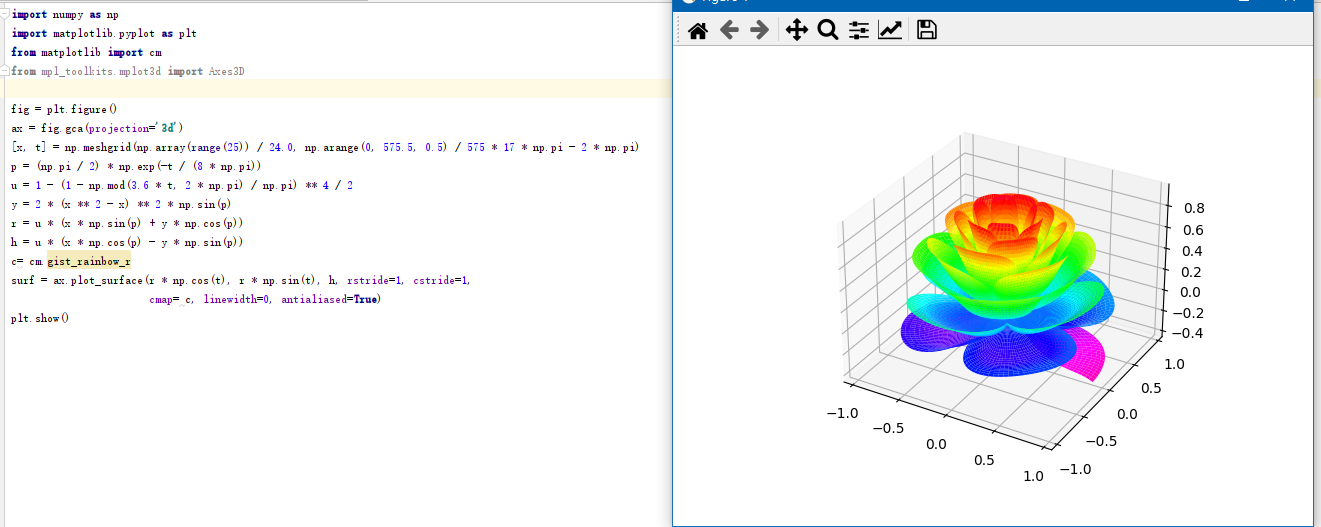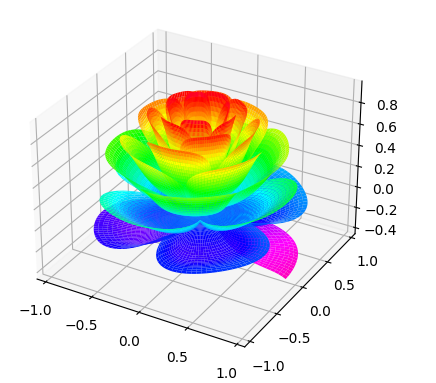import numpy as np
import matplotlib.pyplot as plt
from matplotlib import cm
from mpl_toolkits.mplot3d import Axes3D

fig = plt.figure()
ax = fig.gca(projection='3d')
[x, t] = np.meshgrid(np.array(range(25)) / 24.0, np.arange(0, 575.5, 0.5) / 575 * 17 * np.pi - 2 * np.pi)
p = (np.pi / 2) * np.exp(-t / (8 * np.pi))
u = 1 - (1 - np.mod(3.6 * t, 2 * np.pi) / np.pi) ** 4 / 2
y = 2 * (x ** 2 - x) ** 2 * np.sin(p)
r = u * (x * np.sin(p) + y * np.cos(p))
h = u * (x * np.cos(p) - y * np.sin(p))
c= cm.gist_rainbow_r
surf = ax.plot_surface(r * np.cos(t), r * np.sin(t), h, rstride=1, cstride=1,
cmap= c, linewidth=0, antialiased=True)
plt.show()


三、玫瑰花绘制—粉红色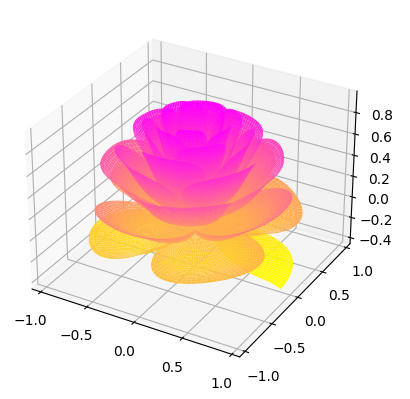import numpy as np
import matplotlib.pyplot as plt
from matplotlib import cm
from mpl_toolkits.mplot3d import Axes3D

fig = plt.figure()
ax = fig.gca(projection='3d')
[x, t] = np.meshgrid(np.array(range(25)) / 24.0, np.arange(0, 575.5, 0.5) / 575 * 17 * np.pi - 2 * np.pi)
p = (np.pi / 2) * np.exp(-t / (8 * np.pi))
u = 1 - (1 - np.mod(3.6 * t, 2 * np.pi) / np.pi) ** 4 / 2
y = 2 * (x ** 2 - x) ** 2 * np.sin(p)
r = u * (x * np.sin(p) + y * np.cos(p))
h = u * (x * np.cos(p) - y * np.sin(p))
c= cm.get_cmap('spring_r')
surf = ax.plot_surface(r * np.cos(t), r * np.sin(t), h, rstride=1, cstride=1,
cmap= c, linewidth=0, antialiased=True)
plt.show()

四、玫瑰花绘制—红色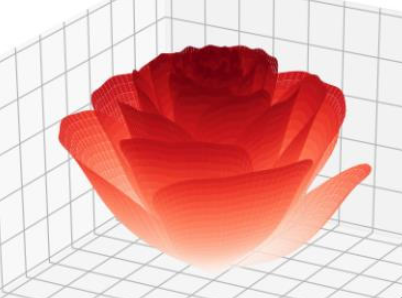# 省略了头文件，可以在之前的博客里看到
import numpy as np
import matplotlib.pyplot as plt
from mpl_toolkits.mplot3d import Axes3D
fig = plt.figure()
ax = fig.gca(projection='3d')
# 将相位向后移动了6*pi
[x, t] = np.meshgrid(np.array(range(25)) / 24.0, np.arange(0, 575.5, 0.5) / 575 * 20 * np.pi + 4*np.pi)
p = (np.pi / 2) * np.exp(-t / (8 * np.pi))
# 添加边缘扰动
change = np.sin(15*t)/150
# 将t的参数减少，使花瓣的角度变大
u = 1 - (1 - np.mod(3.3 * t, 2 * np.pi) / np.pi) ** 4 / 2 + change
y = 2 * (x ** 2 - x) ** 2 * np.sin(p)
r = u * (x * np.sin(p) + y * np.cos(p))
h = u * (x * np.cos(p) - y * np.sin(p))
c= plt.get_cmap('Reds')
surf = ax.plot_surface(r * np.cos(t), r * np.sin(t), h, rstride=1, cstride=1,
cmap= c, linewidth=0, antialiased=True)
plt.show()


五、桃花绘制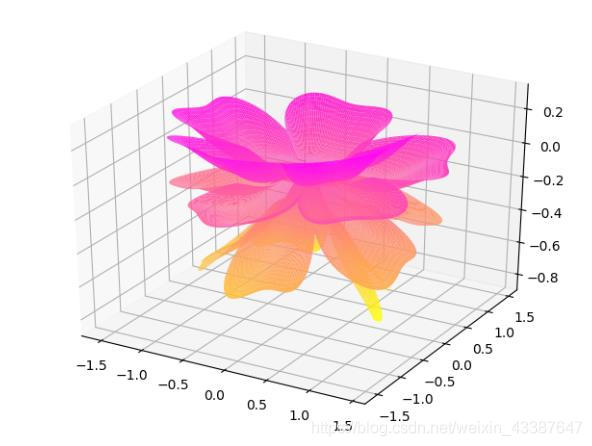import numpy as np
import matplotlib.pyplot as plt
from mpl_toolkits.mplot3d import Axes3D
fig = plt.figure()
ax = fig.gca(projection='3d')
[x, t] = np.meshgrid(np.array(range(25)) / 24.0, np.arange(0, 575.5, 0.5) / 575 * 6 * np.pi - 4*np.pi)
p = (np.pi / 2) * np.exp(-t / (8 * np.pi))
change = np.sin(10*t)/20
u = 1 - (1 - np.mod(5.2 * t, 2 * np.pi) / np.pi) ** 4 / 2 + change
y = 2 * (x ** 2 - x) ** 2 * np.sin(p)
r = u * (x * np.sin(p) + y * np.cos(p)) * 1.5
h = u * (x * np.cos(p) - y * np.sin(p))
c= plt.get_cmap('spring_r')
surf = ax.plot_surface(r * np.cos(t), r * np.sin(t), h, rstride=1, cstride=1,
cmap= c, linewidth=0, antialiased=True)
plt.show()


———————————————— 参考文章 https://blog.csdn.net/weixin_43387647/article/details/88973568
搬运不易，路过的各位大佬请点个赞
添加了import的包，可直接运行
展开全文python
• Graphics 类相当于一个画布，每个 Swing 组件都通过 Graphics 对象来绘制显示。绘图的原点位于组件的左上角，如下图所示: Graphics类中常用的绘制相关方法： ○ 参数 / 设置: // 创建...
本文链接: https://blog.csdn.net/xietansheng/article/details/55669157
Java Swing 图形界面开发（目录）
1. Graphics 绘图画布
Graphics 类相当于一个画布，每个 Swing 组件都通过 Graphics 对象来绘制显示。绘图的原点位于组件的左上角，如下图所示: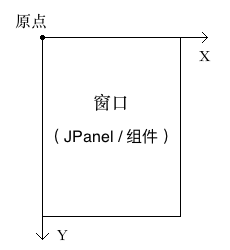Graphics类中常用的绘制相关方法：
○ 参数 / 设置:
// 创建 Graphics 的副本
Graphics create()
// 回收 Graphics
void dispose()

// 设置画笔颜色
void setColor(Color c)
// 擦除某一区域（擦除后显示背景色）
void clearRect(int x, int y, int width, int height)

1、线段 / 折线:
// 绘制一条线段（如果两点为同一点，则绘制点）
void drawLine(int x1, int y1, int x2, int y2)
// 根据给定的多个点坐标绘制折线
void drawPolyline(int xPoints[], int yPoints[], int nPoints)

2、矩形 / 多边形:
// 绘制一个矩形（空心）
void drawRect(int x, int y, int width, int height)
// 填充一个矩形（实心）
void fillRect(int x, int y, int width, int height)

// 绘制一个圆角矩形
void drawRoundRect(int x, int y, int width, int height, int arcWidth, int arcHeight)
// 填充一个圆角矩形
void fillRoundRect(int x, int y, int width, int height, int arcWidth, int arcHeight)

// 绘制一个3D矩形
void draw3DRect(int x, int y, int width, int height, boolean raised)
// 填充一个3D矩形
void fill3DRect(int x, int y, int width, int height, boolean raised)

// 根据给定的多个点坐标绘制一个多边形（首尾相连）
void drawPolygon(int xPoints[], int yPoints[], int nPoints)
// 根据给定的多个点坐标填充一个多边形（首尾相连）
void fillPolygon(int xPoints[], int yPoints[], int nPoints)

3、圆弧 / 扇形:
// 绘制一个圆弧（弧线）
void drawArc(int x, int y, int width, int height, int startAngle, int arcAngle)
// 填充一个圆弧（扇形）
void fillArc(int x, int y, int width, int height, int startAngle, int arcAngle)

4、椭圆:
// 绘制一个椭圆
void drawOval(int x, int y, int width, int height)
// 填充一个椭圆
void fillOval(int x, int y, int width, int height)

5、图片
/*
* 先读取图片
*/
// 方法一: 通过 java.awt.Toolkit 工具类来读取本地、网络 或 内存中 的 图片（支持 GIF、JPEG 或 PNG）
Image image = Toolkit.getDefaultToolkit().getImage(String filename);
Image image = Toolkit.getDefaultToolkit().getImage(URL url);
Image image = Toolkit.getDefaultToolkit().createImage(byte[] imageData);

// 方法二: 通过 javax.imageio.ImageIO 工具类读取本地、网络 或 内存中 的 图片（BufferedImage 继承自 Image）

/*
* PS_01: 图片宽高: BufferedImage 可以通过 bufImage.getWidth() 和 bufImage.getHeight() 方法直接获取图片的宽高;
*                 Image 获取宽高需要另外传入一个 ImageObserver 参数。
*
* PS_02: 图片裁剪: BufferedImage 通过 bufImage.getSubimage(int x, int y, int w, int h) 方法可以截取图片的
*                 任意一部分，返回一个新的 BufferedImage 实例。
*
* PS_03: 图片缩放: Image 通过 image.getScaledInstance(int width, int height, int hints) 方法可以对图片进行
*                 缩放，返回新的一个 Image 实例。
*/

// 绘制一张图片（所有组件类实现了 ImageObserver 接口，即组件实例即为 ImageObserver）
boolean drawImage(Image image, int x, int y, int width, int height, ImageObserver observer)

6、文本
// 设置字体（字体、样式、大小）
void setFont(Font font)
// 绘制一段文本，其中 (x, y) 坐标指的是文本序列的 左下角 的位置
void drawString(String str, int x, int y)

2. Graphics2D
在 Swing 组件的实际绘制中通常使用的是 Graphics 的一个子类 Graphics2D, 该类中提供了更为丰富的接口来绘制更为复杂的需求。
Graphics2D类中的部分方法：
1、参数 / 设置
// 设置背景（擦除后显示该背景）
void setBackground(Color color)
// 设置笔画的轮廓特性（如画笔宽度、实线、虚线等）
void setStroke(Stroke s)

2、绘制结果的变换
// 平移
void translate(int x, int y)
// 旋转
void rotate(double theta, double originX, double originY)
// 缩放
void scale(double sx, double sy)

// 将图片经过指定变换后绘制显示
boolean drawImage(Image image, AffineTransform xform, ImageObserver obs)

3. 自定义组件, 绘图演示
package com.xiets.drawdemo;

import javax.swing.*;
import java.awt.*;

public class Main {

public static void main(String[] args) {
/*
* 在 AWT 的事件队列线程中创建窗口和组件, 确保线程安全,
* 即 组件创建、绘制、事件响应 需要处于同一线程。
*/
EventQueue.invokeLater(new Runnable() {
@Override
public void run() {
// 创建窗口对象
MyFrame frame = new MyFrame();
// 显示窗口
frame.setVisible(true);
}
});
}

/**
* 窗口
*/
public static class MyFrame extends JFrame {

public static final String TITLE = "Java图形绘制";

public static final int WIDTH = 250;
public static final int HEIGHT = 300;

public MyFrame() {
super();
initFrame();
}

private void initFrame() {
// 设置 窗口标题 和 窗口大小
setTitle(TITLE);
setSize(WIDTH, HEIGHT);

// 设置窗口关闭按钮的默认操作(点击关闭时退出进程)
setDefaultCloseOperation(WindowConstants.EXIT_ON_CLOSE);

// 把窗口位置设置到屏幕的中心
setLocationRelativeTo(null);

// 设置窗口的内容面板
MyPanel panel = new MyPanel(this);
setContentPane(panel);
}

}

/**
* 内容面板
*/
public static class MyPanel extends JPanel {

private MyFrame frame;

public MyPanel(MyFrame frame) {
super();
this.frame = frame;
}

/**
* 绘制面板的内容: 创建 JPanel 后会调用一次该方法绘制内容,
* 之后如果数据改变需要重新绘制, 可调用 updateUI() 方法触发
* 系统再次调用该方法绘制更新 JPanel 的内容。
*/
@Override
protected void paintComponent(Graphics g) {
super.paintComponent(g);

// 重新调用 Graphics 的绘制方法绘制时将自动擦除旧的内容

/* 自行打开下面注释查看各绘制效果 */

// 1. 线段 / 折线
drawLine(g);

// 2. 矩形 / 多边形
// drawRect(g);

// 3. 圆弧 / 扇形
// drawArc(g);

// 4. 椭圆
// drawOval(g);

// 5. 图片
// drawImage(g);

// 6. 文本
// drawString(g);
}

/**
* 1. 线段 / 折线
*/
private void drawLine(Graphics g) {
frame.setTitle("1. 线段 / 折线");

// 创建 Graphics 的副本, 需要改变 Graphics 的参数,
// 这里必须使用副本, 避免影响到 Graphics 原有的设置
Graphics2D g2d = (Graphics2D) g.create();

// 抗锯齿
g2d.setRenderingHint(RenderingHints.KEY_ANTIALIASING, RenderingHints.VALUE_ANTIALIAS_ON);
// 设置画笔颜色
g2d.setColor(Color.RED);

// 1. 两点绘制线段: 点(20, 50), 点(200, 50)
g2d.drawLine(50, 50, 200, 50);

// 2. 多点绘制折线: 点(50, 100), 点(100, 130), 点(150, 70), 点(200, 100)
int[] xPoints = new int[] { 50, 100, 150, 200 };
int[] yPoints = new int[] { 100, 120, 80, 100 };
int nPoints = 4;
g2d.drawPolyline(xPoints, yPoints, nPoints);

// 3. 两点绘制线段（设置线宽为5px）: 点(50, 150), 点(200, 150)
BasicStroke bs1 = new BasicStroke(5);       // 笔画的轮廓（画笔宽度/线宽为5px）
g2d.setStroke(bs1);
g2d.drawLine(50, 150, 200, 150);

// 4. 绘制虚线: 将虚线分为若干段（ 实线段 和 空白段 都认为是一段）, 实线段 和 空白段 交替绘制,
//             绘制的每一段（包括 实线段 和 空白段）的 长度 从 dash 虚线模式数组中取值（从首
//             元素开始循环取值）, 下面数组即表示每段长度分别为: 5px, 10px, 5px, 10px, ...
float[] dash = new float[] { 5, 10 };
BasicStroke bs2 = new BasicStroke(
1,                      // 画笔宽度/线宽
BasicStroke.CAP_SQUARE,
BasicStroke.JOIN_MITER,
10.0f,
dash,                   // 虚线模式数组
0.0f
);
g2d.setStroke(bs2);
g2d.drawLine(50, 200, 200, 200);

// 自己创建的副本用完要销毁掉
g2d.dispose();
}

/**
* 2. 矩形 / 多边形
*/
private void drawRect(Graphics g) {
frame.setTitle("2. 矩形 / 多边形");
Graphics2D g2d = (Graphics2D) g.create();

g2d.setRenderingHint(RenderingHints.KEY_ANTIALIASING, RenderingHints.VALUE_ANTIALIAS_ON);
g2d.setColor(Color.GRAY);

// 1. 绘制一个矩形: 起点(30, 20), 宽80, 高100
g2d.drawRect(30, 20, 80, 100);

// 2. 填充一个矩形
g2d.fillRect(140, 20, 80, 100);

// 3. 绘制一个圆角矩形: 起点(30, 150), 宽80, 高100, 圆角宽30, 圆角高30
g2d.drawRoundRect(30, 150, 80, 100, 30, 30);

// 4. 绘制一个多边形(收尾相连): 点(140, 150), 点(180, 250), 点(220, 200)
int[] xPoints = new int[] { 140, 180, 220};
int[] yPoints = new int[] { 150,  250, 200};
int nPoints = 3;
g2d.drawPolygon(xPoints, yPoints, nPoints);

g2d.dispose();
}

/**
* 3. 圆弧 / 扇形
*/
private void drawArc(Graphics g) {
frame.setTitle("3. 圆弧 / 扇形");
Graphics2D g2d = (Graphics2D) g.create();

g2d.setRenderingHint(RenderingHints.KEY_ANTIALIASING, RenderingHints.VALUE_ANTIALIAS_ON);
g2d.setColor(Color.RED);

// 1. 绘制一条圆弧: 椭圆的外切矩形 左上角坐标为(0, 0), 宽100, 高100,
//                弧的开始角度为0度, 需要绘制的角度数为-90度,
//                椭圆右边水平线为0度, 逆时针为正角度, 顺时针为负角度
g2d.drawArc(0, 0, 100, 100, 0, -90);

// 2. 绘制一个圆: 圆的外切矩形 左上角坐标为(120, 20), 宽高为100
g2d.drawArc(120, 20, 100, 100, 0, 360);

g2d.setColor(Color.GRAY);

// 3. 填充一个扇形
g2d.fillArc(80, 150, 100, 100, 90, 270);

g2d.dispose();
}

/**
* 4. 椭圆 (实际上通过绘制360度的圆弧/扇形也能达到绘制圆/椭圆的效果)
*/
private void drawOval(Graphics g) {
frame.setTitle("4. 椭圆");
Graphics2D g2d = (Graphics2D) g.create();

g2d.setRenderingHint(RenderingHints.KEY_ANTIALIASING, RenderingHints.VALUE_ANTIALIAS_ON);
g2d.setColor(Color.RED);

// 1. 绘制一个圆: 圆的外切矩形 左上角坐标为(0, 0), 宽高为100
g2d.drawOval(0, 0, 100, 100);

g2d.setColor(Color.GRAY);

// 2. 填充一个椭圆
g2d.fillOval(120, 100, 100, 150);

g2d.dispose();
}

/**
* 5. 图片
*/
private void drawImage(Graphics g) {
frame.setTitle("5. 图片");
Graphics2D g2d = (Graphics2D) g.create();

// 从本地读取一张图片
String filepath = "demo.jpg";
Image image = Toolkit.getDefaultToolkit().getImage(filepath);

// 绘制图片（如果宽高传的不是图片原本的宽高, 则图片将会适当缩放绘制）
g2d.drawImage(image, 50, 50, image.getWidth(this), image.getHeight(this), this);

g2d.dispose();
}

/**
* 6. 文本
*/
private void drawString(Graphics g) {
frame.setTitle("6. 文本");
Graphics2D g2d = (Graphics2D) g.create();

g2d.setRenderingHint(RenderingHints.KEY_ANTIALIASING, RenderingHints.VALUE_ANTIALIAS_ON);

// 设置字体样式, null 表示使用默认字体, Font.PLAIN 为普通样式, 大小为 25px
g2d.setFont(new Font(null, Font.PLAIN, 25));

// 绘制文本, 其中坐标参数指的是文本绘制后的 左下角 的位置
// 首次绘制需要初始化字体, 可能需要较耗时
g2d.drawString("Hello World!", 20, 60);
g2d.drawString("你好, 世界!", 20, 120);

g2d.dispose();
}

}

}

结果展示: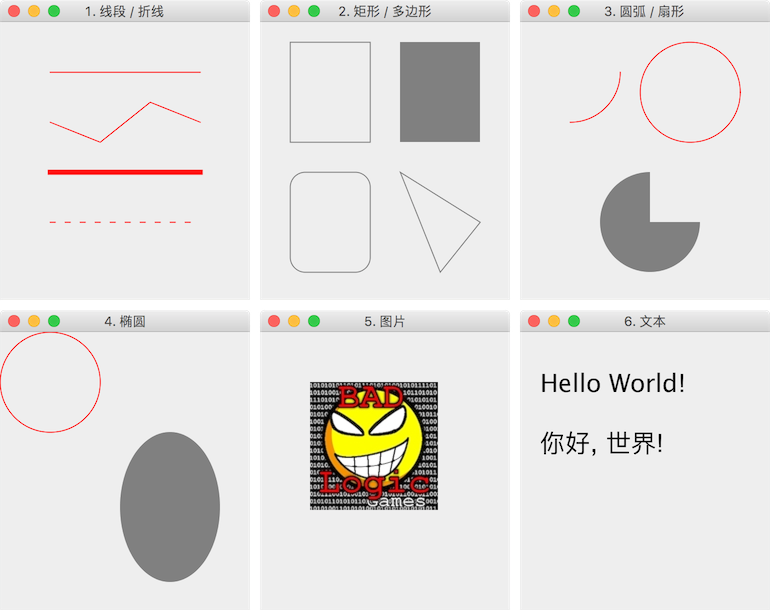4. 把组件上绘制的内容保存为图片
// import javax.imageio.ImageIO;
// import java.awt.image.BufferedImage;

// 获取到需要保存内容的组件（面板）
JPanel panel = (JPanel) frame.getContentPane();

// 创建一个与面板等宽高的缓存图片
BufferedImage image = new BufferedImage(
panel.getWidth(),
panel.getHeight(),
BufferedImage.TYPE_INT_ARGB
);

// 获取缓存图片的画布
Graphics2D g2d = image.createGraphics();

// 把面板的内容画到画布中
panel.paint(g2d);

try {
// 把缓存图片保存到本地文件
ImageIO.write(image, "png", new File("panel.png"));
} catch (Exception e) {
e.printStackTrace();
}

扩展: Java图片操作 — 图片的读取、绘制、缩放、裁剪、保存
展开全文java java绘图 Graphics Graphics2D swing绘图
• ## 自己绘制菜单

千次下载 热门讨论 2015-06-11 13:03:18
• ## 绘制UML各种图详解

万次阅读 多人点赞 2018-01-30 14:08:48
一、前言 在软件开发过程中会经常整理文档，不是所有的信息都可以通过文字来表达，而换成图之后会更加的直观、有效，其中频繁使用的就是UML图。...本文中的UML图都是用visio 绘制，source code展示是Ja...
来源：绘制UML各种图详解_私房菜-CSDN博客_uml图

一、前言
在软件开发过程中会经常整理文档，不是所有的信息都可以通过文字来表达，而换成图之后会更加的直观、有效，其中频繁使用的就是UML图。结合自己实践总结一下，希望对大家都能有所帮助。

本文中的UML图都是用visio 绘制，source code展示是Java 语言。

二、UML简介

UML图包括两个范畴：结构图和行为图。
结构图的目的是显示建模系统的静态结构。它包括类、组件和对象。例如UML静态结构图。
行为图的目的是显示系统中对象的动态行为。它包括对象的方法、协作和活动之类的内容。例如UML示例图、UML活动图、UML序列图。

三、UML类图
通过UML类图可以将类之间的所有关系（泛化、实现、关联、聚合、组合、依赖）都能具体的体现出来。
1、类图的表示
类的 UML 表示是一个长方形，垂直地分为三个区，如下图所示。顶部区域显示类的名字。中间的区域列出类的属性。底部的区域列出类的操作。在一个类图上画一个类元素时，你必须要有顶端的区域，下面的二个区域是可选择的（当图描述仅仅用于显示分类器间关系的高层细节时，下面的两个区域是不必要的）。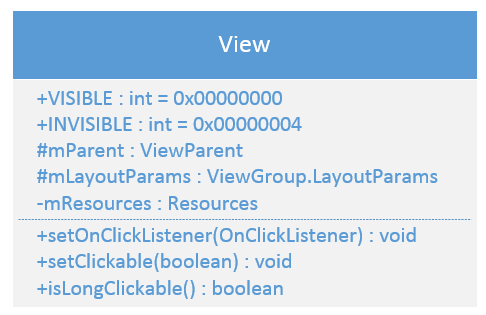顶端是类名，中间列出的是类的属性。底部列出的是类的操作。在绘制类图的时候，顶端部分是必须的，其他两个部分可选。
类名：如果是抽象类，采用斜体。
类属性列表：可见性 + 属性名 + 冒号 + 属性类型 + （等号 + 默认值）
属性的可见性下面会说明；属性的类型看属性而定，可以是int，可以是boolean；有的属性可能会有默认值，在类型之后加等号和默认值。
类方法列表：可见性 + 函数名 + (参数列表) + 冒号 + 返回值类型
可见性：

标志可见性类型+public-private#protected~package

2、继承的表示

为了在一个类图上建模继承，从子类（要继承行为的类）拉出一条闭合的单键头（或三角形）的实线指向超类。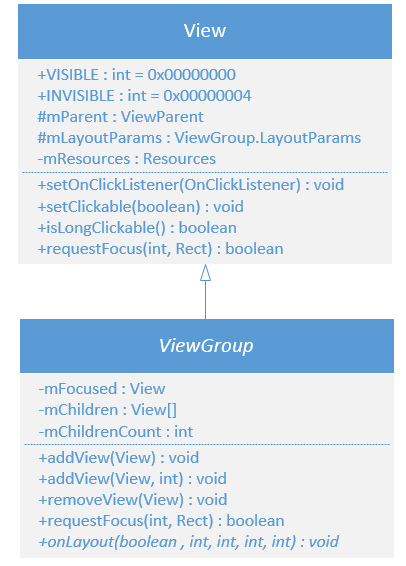ViewGroup 和 方法onLayout 用的是斜体，说明ViewGroup 是抽象类，方法onLayout 是抽象的。

3、接口的表示
一个类和一个接口不同：一个类可以有它形态的真实实例，然而一个接口必须至少有一个类来实现它。在 UML 中，一个接口被认为是类建模元素的特殊化。因此，接口就象类那样绘制，但是长方形的顶部区域也有文本“interface”。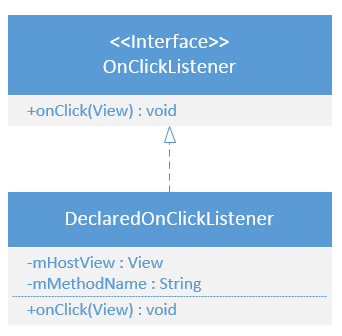对于接口，如果用<< 和 >>将字符串interface包起来，表示这是个接口类。并且用虚线带三角形的箭头从实现类指向Interface 类。

4、关联的表示
（1）双向关联
在两个类之间用实线连接，表示两个类彼此都知道它们之间的联系。在实线的上端写上关联关系，例如 has；在实线的下端写上可能的多重值，多重值表示如下：

表示含义0..10个或者1个1只能1个0..*0个或者多个*0个或者多个1..*1个或者多个3只能3个0..30至3个3..133至13个
（2）单向关联
单向关联是用一个实线带一个开放的箭头，表示一端知道箭头指向的另一端。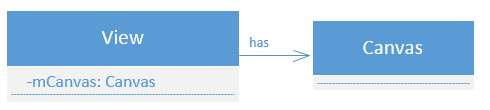上图中View 中包含Canvas 类，而Canvas 中不需要包含View。

5、聚合的表示
聚合是一种特别类型的关联，用于描述“总体到局部”的关系。在基本的聚合关系中，部分类的生命周期独立于整体类的生命周期。用一个带空心的菱形的实线表示，菱形指向的是整体的类。
例如，汽车和轮子的关系：聚合与单向关联的区别在于：单项的关联可能只是一个简单的“包含、引用”关系，关联和被关联类之间在逻辑概念上不一定有紧密的联系，而聚合则不同，它表示的是一种内在关系紧密，相互依存，相互包含的概念，其中的一部分是构成另外一部分的不可或缺的成分。

6、组合的表示
组合聚合关系是聚合关系的另一种形式，但是子类实例的生命周期依赖于父类实例的生命周期。
用一个带实心的菱形的实线表示，菱形指向的是整体的类。
组合跟聚合有很大的不同，聚合的部分的生命周期独立于整体，整体结束那不影响部分。而组合是部分的生命周期依赖与整体，整体结束，部分没有必要存在。就想公司和部分，公司都不存在了，那部门就没有存在的必要了，解散了算。
同样用View 为例：其中的DeclaredOnclickListener 类是View的一部分，而且是依赖View的生命周期。

7、依赖的表示
依赖（Dependency）关系是一种使用关系，特定事物的改变有可能会影响到使用该事物的其他事物，在需要表示一个事物使用另一个事物时使用依赖关系，大多数情况下依赖关系体现在某个类的方法使用另一个类的对象作为参数。在UML中，依赖关系用带箭头的虚线表示，由依赖的一方指向被依赖的一方。

依赖关系通常通过三种方式来实现：
将一个类的对象作为另一个类中方法的参数在一个类的方法中将另一个类的对象作为其对象的局部变量在一个类的方法中调用另一个类的静态方法

关联关系、聚合、组合之间的区别：
关联关系跟突出的是has，只是has的关系，可以通过这一层关系调用到另一个对象中的某个方法。
聚合、组合更突出“整体-部分”的关系，不单单是“拥有”，部分类是构成整体类的一部分。
对于聚合、组合，区别就在于部分类的生命周期如何，如果部分类还可以独立存在，那属于聚合关系；如果部分类不能独立存在，那属于组合的关系。

后话：
今天看到@fix_bugs 朋友的热心留言，还是很有感触了，技术是没有界限的，大家只有将好的东西分享出来，我们效率才会更高，技术才能更强大，祖国才会更强大。 因为viso license 的问题，我目前大部分用drawio，Flowchart Maker & Online Diagram Software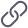https://app.diagrams.net/ 推荐大家使用！！ 大家有什么好的，也请不吝分享出来，感谢！！


展开全文• ## 用python绘制散点图

万次阅读 多人点赞 2018-07-13 21:41:53
今天下午学习了如何使用python绘制简单的散点图，写成博客分享一下。 在python中画散点图主要是用matplotlib模块中的scatter函数，先来看一下scatter函数的基本信息。 网址为：点击打开链接 可以看到scatter中有很多...
在python中画散点图主要是用matplotlib模块中的scatter函数，先来看一下scatter函数的基本信息。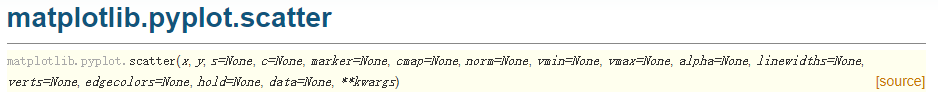网址为：点击打开链接
可以看到scatter中有很多参数，经常使用的参数主要有以下几个：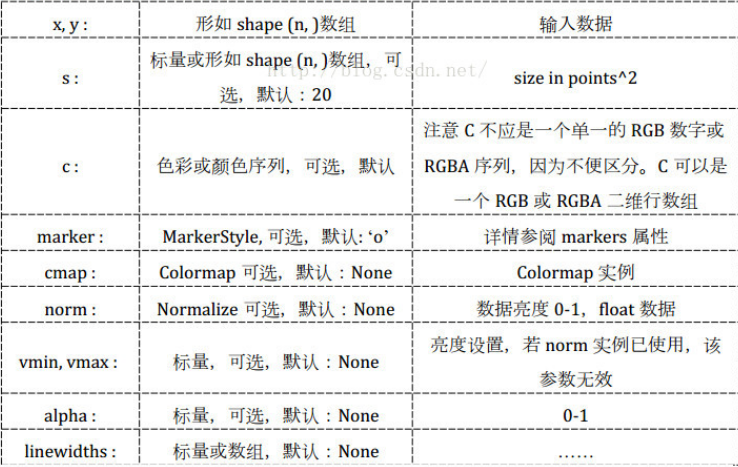c：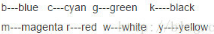marker: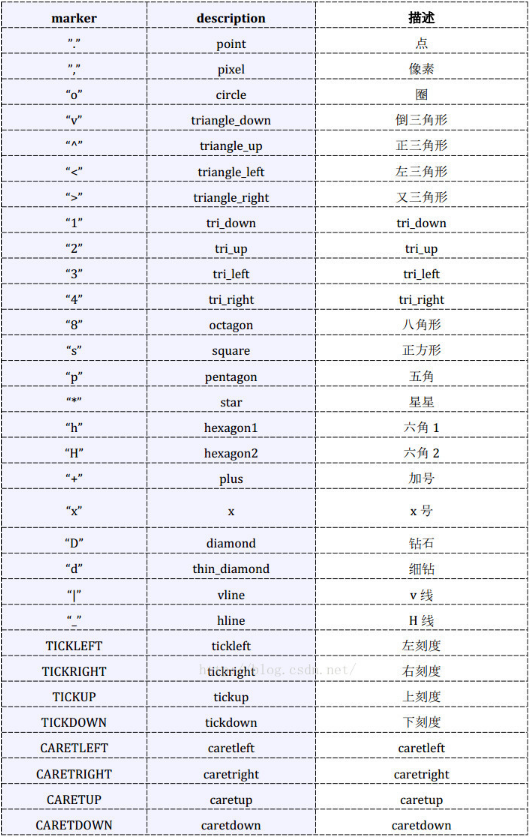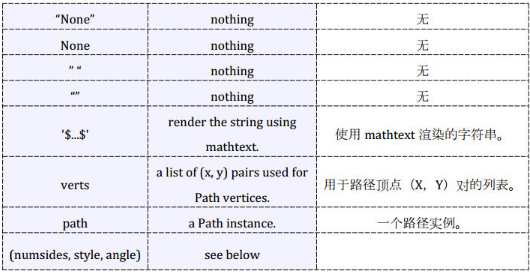数据、代码和绘制的图如下。
数据（取第一列作为x，取第四列作为y）截图：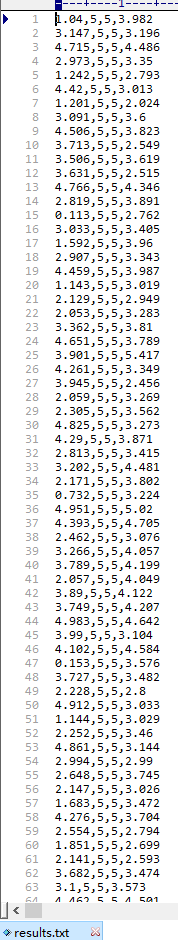代码如下：
import matplotlib.pyplot as plt
import numpy as np

# 定义画散点图的函数
def draw_scatter(n, s):
"""
:param n: 点的数量，整数
:param s:点的大小，整数
:return: None
"""
# 加载数据
# 通过切片获取横坐标x1
x1 = data[:, 0]
# 通过切片获取纵坐标R
y1 = data[:, 3]
# 横坐标x2
x2 = np.random.uniform(0, 5, n)
# 纵坐标y2
y2 = np.array( * n)
# 创建画图窗口
fig = plt.figure()
# 将画图窗口分成1行1列，选择第一块区域作子图
# 设置标题
ax1.set_title('Result Analysis')
# 设置横坐标名称
ax1.set_xlabel('gamma-value')
# 设置纵坐标名称
ax1.set_ylabel('R-value')
# 画散点图
ax1.scatter(x1, y1, s=s, c='k', marker='.')
# 画直线图
ax1.plot(x2, y2, c='b', ls='--')
# 调整横坐标的上下界
plt.xlim(xmax=5, xmin=0)
# 显示
plt.show()

# 主模块
if __name__ == "__main__":
# 运行
draw_scatter(n=2000, s=20)
绘制的图如下：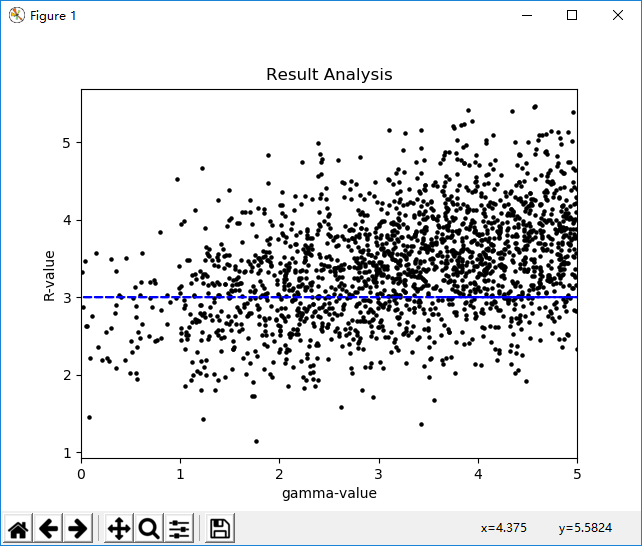展开全文散点图 Python scatter
•c#
• 本人也是前端小白，正在努力学习的路上奔跑~最近看到一位博主大大的绘制星空博文，加上最近对面向对象编程思想的理解，也照葫芦画瓢复现了一个demo，顺便熟悉一下canvas的一些API。 源代码 <!DOCTYPE html> &...javascript html5 es6 css3
• ## 利用R绘制漂亮的中国地图

万次阅读 多人点赞 2018-09-22 11:16:24地图
• ## C# 绘制实时曲线包括绘制坐标轴

千次下载 热门讨论 2014-08-06 02:08:35
C# 绘制实时曲线及坐标轴，实现实时的图像处理操作。
• 1. 概要 体绘制（Volume rendering）在医疗领域有着重要作用，...本文从体绘制的几个研究类别着手，介绍一些体绘制方法，能够让初涉该领域的研究者对体绘制过程有所了解。 图1 Rain-Water (https://draemm.li/v...
• 使用openGL绘制正方体； 面1：使用简单颜色绘制，颜色为绿色； 面2：使用简单颜色绘制，颜色为渐变色； 面3：绘制一维纹理； 面4：绘制二维纹理； 面5：光照绘制，黄色； 面6：光照绘制，橘色； 绘制效果如下： ...
• 在C#绘制中国象棋棋盘是C#程序设计中GDI+的一个重要组成部分。这也是非常考验编程技巧的操作。在绘制之前首先要对棋盘有一个完整的认识。下面是完成后的输出图案。 ##1.在制作过程中用到了背景图片如下： ##2.棋盘...程序人生 经验分享 c#
• C#绘制坐标轴绘制坐标轴绘制坐标轴绘制坐标轴C#C#绘制坐标轴绘制坐标轴绘制坐标轴绘制坐标轴C#绘制坐标轴绘制坐标轴绘制坐标轴绘制坐标轴C#绘制坐标轴绘制坐标轴绘制坐标轴绘制坐标轴C# 坐标轴
• Open3D绘制3D坐标，绘制点云 # -*-coding: utf-8 -*- """ @Project: PyKinect2-OpenCV @File : open3d_test.py @Author : panjq @E-mail : pan_jinquan@163.com @Date : 2019-10-10 09:49:27 ""...Open3D
• ## MATLAB05:绘制高级图表

万次阅读 多人点赞 2019-11-16 16:22:30
文章目录MATLAB05:绘制高级图表二维图表折线图对数坐标系图线双y轴图线极坐标图线统计图表三维图表 MATLAB05:绘制高级图表 二维图表 折线图 函数 图形描述 loglog() x轴和y轴都取对数坐标 semilogx() x轴...MATLAB绘图
• 动态绘制曲线。 可以非常直观看出数据的走 向。Qt界面
• 通过重写UIView的drawRect方法进行绘制使用，如绘制文本字符、绘制图片、绘制图形等。 注意事项： 1、设置frame的属性，或调用setNeedsDisplay时才会调用drawRect方法。 2、在绘制过程中 （1）针对实际情况获取图形...
• 那么本篇文章中，我们将继续对View进行深入探究，看一看它的绘制流程到底是什么样的。如果你还没有看过我的上一篇文章，可以先去阅读 Android LayoutInflater原理分析，带你一步步深入了解View(一) 。 相信每个...Android View onMeasure onLayout onDraw
• 概述 本人使用Cadence 17.4版本，在这做下笔录，绘制板边与限制布线区域方法。 一、绘制板边 二、设置布线区域

千次阅读 2020-11-28 15:26:55
• 对于初学者来说，很容易被这官网上众多的图表类型给吓着了，由于种类太多，几种图表的绘制方法很有可能会混淆起来。 因此，在这里，我特地总结了六种常见的基本图表类型，你可以通过对比学习，打下坚实的基础。 01...python
• ## UI绘制优化

千次阅读 2019-08-28 15:39:26
文章目录CPU与GPU过度绘制概念：过度绘制出现的原因过渡绘制查看过度绘制优化hierarchy view 查看布局结构图 CPU与GPU CPU 的任务繁多，做逻辑计算外，还要做内存管理、显示操作，因此 在实际运算的时候性能会大打...CPU与GPU viewstub
• ## Matlab绘制折线图详细方法

万次阅读 多人点赞 2018-04-11 12:17:55
Matlab绘制折线图属于非常基本的功能，首先给出一个示例代码，有详细的解释，可以根据自己需求进行修改。 x=1:1:5;%x轴上的数据，第一个值代表数据开始，第二个值代表间隔，第三个值代表终止 a=[203.024,113.857...matlab
• python绘制蟒蛇，绘制五彩蟒蛇 1.用Python绘制蟒蛇 from turtle import * setup(650,350,200,200) penup() fd(-250) pendown() pensize(25) pencolor("purple") seth(-40) for i in range(4): circle(40,80) ...python
• ## PCB绘制

千次阅读 2019-04-24 19:39:01
之前有学过PCB的绘制，大二上学期的时候吧，当时只画了一块旋转LED的板子，后来就没再画过了，然后现在就导致自己有点儿忘记了，最近想自己做个东西出来，用到了PCB绘制，就再开一篇博客，记录一下具体的过程吧，也...
• this.draw.finishDrawing() Stop drawing and add the sketch feature to the target layer. The module:ol/interaction/Draw~...可以通过键盘快捷键操作，结束draw绘制，将当前绘制的图形添加到目标图层中。 ...javascript OpenLayers
• matplotlib绘制直方图、条形图和饼图 matplotlib是一个非常强大的Python第三方绘图包，可以绘制非常多非常漂亮的图形。matplotlib.pyplot提供了一个类Matlab的函数式编程环境。通常的绘图任务，都可以通过...
• ## OpenCV-绘制同心圆

千次阅读 多人点赞 2021-08-30 15:56:55opencv c++
• 经过寻找，找到了几个在线绘制函数图像的网址，可以不用matlab和geogebra软件绘制了。 数学函数图像： 第一个：Desmos 首推 第二个：fooplot  可以绘制分段函数比如： (x&gt;=1)x*3/(3*x+1) (x&lt;1......